Periodic Functions of wavelength λ using the modulus function:

Mod is a very useful function that can be used to force objects to be periodic.  Mod[x,λ] return that part of x that lies within 0 and λ.  Or, in other words if we map the real line x to a circle with circumference λ, then Mod[x,λ] returns were x is mapped onto the circle.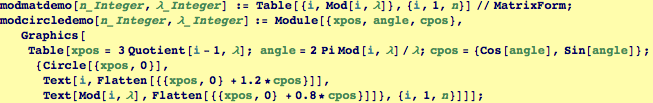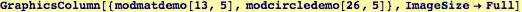Boomerang uses Mod to force a function, f, with a single argument, x, to be periodic with wavelength λ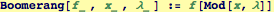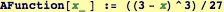The following step uses Boomerang to produce a periodic repetition of AFunction over the range 0 < x < 6: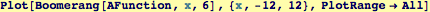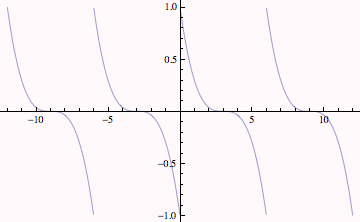Created by Wolfram Mathematica 6.0  (24 October 2007)